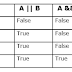Operators are special symbols that perform specific operations on one, two, or three operands, and then return a resultThere are different types of operators are available in java which are given below:
1. Arithmetic Operators.
2. Unary Operators.
3. Assignment Operators.
4. Relational Operators.
5. Logical Operators.
6. BitWise Operators.
7. Ternary Operators.
8. InstanceOf Operators.

## Logical Operators in Java:

Logical Operators in Java works on boolean operands. It is also known as Boolean Logical Operators. It operates on two boolean values, which return boolean values(true/false) as a result. There are basically three types of logical operators used in Java.

1. Logical AND (&&) Operator: This operator returns true if both the conditions are true.

2. Logical OR (||) Operator: This operator returns true if at least one condition is true.

3. Logical Not (!) Operator: This operator reverses the value of the operand. If the value is true, then it returns false, and if it is false, then it returns true.

## Example of Logical Operators:

``````
class LogicalOperator {
public static void main(String[] args) {
//Variables Definition and Initialization
boolean b1 = true, b2 = false;

//Logical AND Operation
System.out.println("b1 && b2 --> " + (b1 && b2));

//Logical OR Operation
System.out.println("b1 || b2 --> " + (b1 || b2) );

//Logical Not Operation
System.out.println("!(b1 && b2) --> " + !(b1 && b2));

}
}
```
```

Output:

You may also like these related posts:
2. Assignment Operators in Java with example.
3. Unary Operators in Java with example.
4. Arithmetic Operators in Java with example.
5. Java Hello World program.
6. How to set path in java.
7. Variables in Java with example
8. Data types in Java.
9. Top 100 java interview question and answer.

Logical Operators in Java with ExampleReviewed by Prashant Srivastava on December 15, 2019 Rating: 5